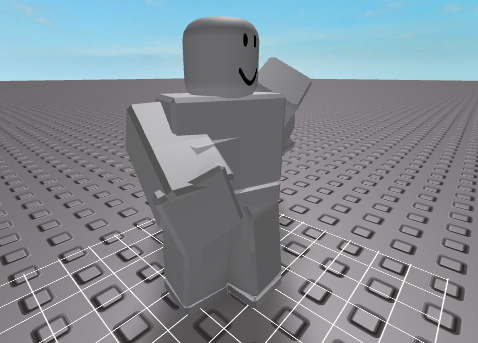# Rotating An R15 Joint Based On The Hand Rotation

I am working on an R6 to R15 converter with basic Inverse Kinematics. The problem I have is that I need to rotate the arm based on the hand, but I am not sure how since I am not that strong in vector math. Here is an example of my old solver which didn’t work in a lot of cases to give an idea:

Included is a file with this model:

The red part is the CFrame of the shoulder, the blue part is the center of the arm, and the green part is the CFrame of the hand. The arc handles represents that axis of rotation to spin to rotate the arm. When run, a joint part and 2 connecting parts will be shown to visualize it.
File: Appendage Rotator.rbxl (15.4 KB)

I am looking for advise on how I solve this, or an actual solution under the “GetHandRotation()” function.

1 Like

This probably isn’t the most efficient way to do this, but it works and it’s a starting point:

``````local function isZero(vector)
return vector.x == 0 and vector.y == 0 and vector.z == 0
end

local function makePerpendicular(axis, forward, up)
local temp = axis:Cross(forward)
if isZero(temp) then  -- backup vector for if forward is parallel to axis
if axis:Dot(forward) > 0 then
up = -up
end
temp = axis:Cross(up)
end
return temp:Cross(axis).Unit, temp
end

--This returns the needed rotation for the hand.
local function GetHandRotation()
local handCFrameInUpperArmSpace = UpperArmCenter.CFrame:toObjectSpace(HandCenter.CFrame)
local axisVector = UpperArmCenter.CFrame.upVector

local upperArmFrontVector, upperArmRightVector = makePerpendicular(axisVector, UpperArmCenter.CFrame.lookVector, UpperArmCenter.CFrame.upVector)

local handFrontVector, handRightVector = makePerpendicular(axisVector, HandCenter.CFrame.lookVector, HandCenter.CFrame.upVector)

local angle = math.clamp(upperArmFrontVector:Dot(handFrontVector), -1, 1)

if angle == 1 or angle == -1 then
if (handFrontVector - upperArmFrontVector):isClose(Vector3.new(0, 0, 0), 0.1) then
print("hand angle: 0")
return 0
elseif (handFrontVector + upperArmFrontVector):isClose(Vector3.new(0, 0, 0), 0.1) then
print("hand angle: 180")
return math.pi
end
end

angle = math.acos(angle)

local temp = upperArmFrontVector:Cross(handFrontVector)
if temp:Dot(axisVector) < 0 then
angle = -angle
end

print("hand angle:",math.deg(angle))
return angle
end
``````

The idea is to get an axis, convert the UpperArm and Hand look vectors to be perpendicular to it, then get the difference in the angle between the UpperArm and Hand’s axis-perpendicular vector.

I’m not sure how good of a solution this is. You can try with different axes and see how it works out. It could probably be written in a more efficient manner, too.

It does handle the case that that look vector is parallel to the axis though (using the up vector or down vector as a backup), so that’s nice!

https://gfycat.com/ExhaustedValuableAtlanticridleyturtle

https://gfycat.com/PhysicalPoliticalGalapagosalbatross

The point where the arm is inverted from the expected position is fixed in the above code.

4 Likes

Looks like that works for me. The one thing I will mention is the “return math.pi*2” seems to be the problem with it inverting.

1 Like

After some testing, this proved to be very problematic in certain cases. I probably will just release the V1 of the plugin tonight with “bad IK” as AxisAngle calls it and hope someone releases a good V2.1 Like

I was pretty tired at the time and thought that pi was 90 degrees. I was also tired enough to think that it printing 180 must have meant it was 180.